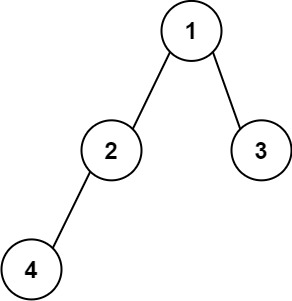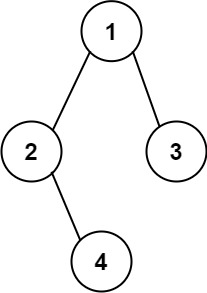# GeetCode Hub

Given the `root` of a binary tree, construct a string consists of parenthesis and integers from a binary tree with the preorder traversing way, and return it.

Omit all the empty parenthesis pairs that do not affect the one-to-one mapping relationship between the string and the original binary tree.

Example 1:```Input: root = [1,2,3,4]
Output: "1(2(4))(3)"
Explanation: Originallay it needs to be "1(2(4)())(3()())", but you need to omit all the unnecessary empty parenthesis pairs. And it will be "1(2(4))(3)"
```

Example 2:```Input: root = [1,2,3,null,4]
Output: "1(2()(4))(3)"
Explanation: Almost the same as the first example, except we cannot omit the first parenthesis pair to break the one-to-one mapping relationship between the input and the output.
```

Constraints:

• The number of nodes in the tree is in the range `[1, 104]`.
• `-1000 <= Node.val <= 1000`

/** * Definition for a binary tree node. * public class TreeNode { * int val; * TreeNode left; * TreeNode right; * TreeNode() {} * TreeNode(int val) { this.val = val; } * TreeNode(int val, TreeNode left, TreeNode right) { * this.val = val; * this.left = left; * this.right = right; * } * } */ class Solution { public String tree2str(TreeNode root) { } }